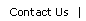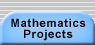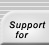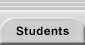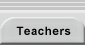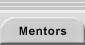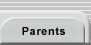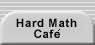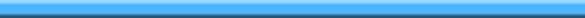Home > Mathematics Projects > Pascal’s Triangle > Resources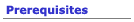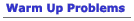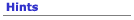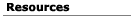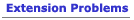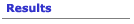## Resources for the Patterns in Pascal’s Triangle Problem

### Pascal’s Triangle Coloring Sheets

Download and print a blank triangle pattern or a pattern with values.

### Modular Arithmetic

Modular Arithmetic at http://www2.makingmath/mathtools/mod/mod.asp.

Divisibility at http://www2.edc.org/mathproblems/problems/htmlProblems/nsDivisibility/nsDivisibility.asp.

### Combinations and Permutations

Education Development Center, Inc., Mathematical Methods: Topics in Discrete and Precalculus Mathematics (see http://www.its-about-time.com/htmls/mm/mm.html for information).

Consult a traditional Precalculus textbook’s chapters on permutations and combinations.

### Pascal’s Triangle

Pascal’s Triangle at http://www2.makingmath/mathtools/pascal/pascal.asp provides information on different ways to derive the triangle, different properties and patterns within the triangle, and Internet sites that generate pictures of the divisibility patterns for further study.

### Fractals

Work your way through Cynthia Lanius’ Fractal Lessons at http://math.rice.edu/~lanius/frac and play with the Snowflake Fractal Generator at http://www.shodor.org/master/fractal/software/Snowflake.html

### Spreadsheets (Excel Format) for Pascal’s Triangle Explorations

CornerPascal.xls. Rows of the triangle are diagonals of the spreadsheet (print it out and turn it 45°!).

 1 1 1 1 1 2 3 4 1 3 6 10 1 4 10 20

SkewPascal.xls. Each row of Pascal’s triangle is a row of the spreadsheet.

 1 1 1 1 2 1 1 3 3 1

SkewPascalModN.xls. Same as the above but with all values displayed mod n. Experiment with different values of the modulus, n.

 1 1 1 1 0 1 1 1 1 1 1 0 0 0 1 1 1 0 0 1 1Translations of mathematical formulas for web display were created by tex4ht. © Copyright 2003 Education Development Center, Inc. (EDC)